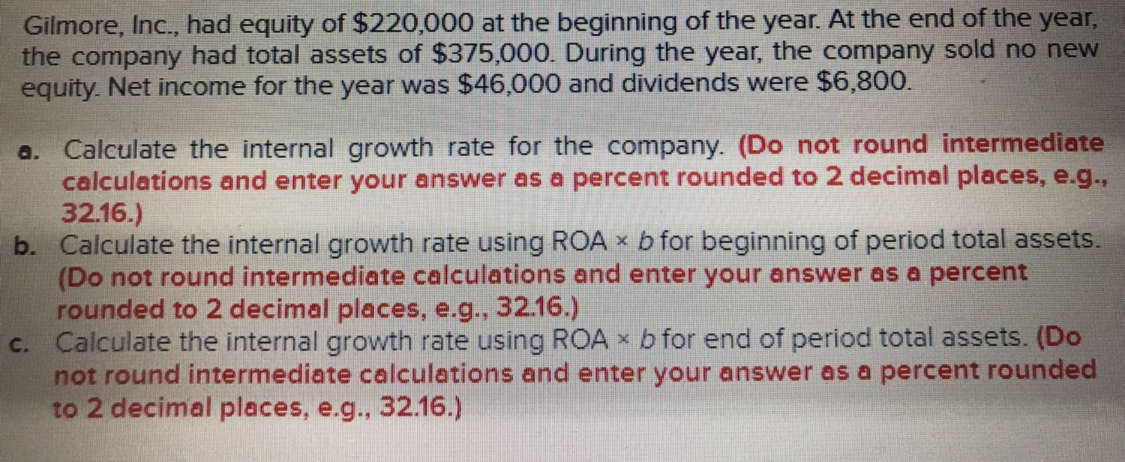# Gilmore, Inc., had equity of \$220,000 at the beginning of the year. At the end of the yearthe company had total assets of \$375,000. During the year, the company sold no newequity. Net income for the year was \$46,000 and dividends were \$6,800.a. Calculate the internal growth rate for the company. (Do not round intermediateb. Calculate the internal growth rate using ROA x b for beginning of period total assets.c. Calculate the internal growth rate using ROA x b for end of period total assets. (Docalculations and enter your answer as a percent rounded to 2 decimal places, e.g.,32.16.)(Do not round intermediate calculations and enter your answer as a percentrounded to 2 decimal places, e.g., 32.16.)not round intermediate calculations and enter your answer as a percent roundedto 2 decimal places, e.g., 32.16.)

Questionhelp_outlineImage TranscriptioncloseGilmore, Inc., had equity of \$220,000 at the beginning of the year. At the end of the year the company had total assets of \$375,000. During the year, the company sold no new equity. Net income for the year was \$46,000 and dividends were \$6,800. a. Calculate the internal growth rate for the company. (Do not round intermediate b. Calculate the internal growth rate using ROA x b for beginning of period total assets. c. Calculate the internal growth rate using ROA x b for end of period total assets. (Do calculations and enter your answer as a percent rounded to 2 decimal places, e.g., 32.16.) (Do not round intermediate calculations and enter your answer as a percent rounded to 2 decimal places, e.g., 32.16.) not round intermediate calculations and enter your answer as a percent rounded to 2 decimal places, e.g., 32.16.) fullscreen
check_circle

Step 1

a. Calculation of Internal Growth Rate of the company

To calculate the internal growth rate of the company, the return on assets during the beginning and end, addition to retained earnings and the plowback ratio has to be calculated as shown below,

Step 2

The calculation of return on assets at the beginning and plowback ratio is shown below,

Step 3

Now calculate the internal growth rate...

### Want to see the full answer?

See Solution

#### Want to see this answer and more?

Solutions are written by subject experts who are available 24/7. Questions are typically answered within 1 hour.*

See Solution
*Response times may vary by subject and question.
Tagged in